# C++ Manipulators

The C++ manipulators are stream functions that modify the characteristics of input and output stream. It is used for formating the input and output stream by changing format flags and values for the stream.

The list of manipulator function is located in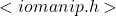header file. You need to include this header to use the manipulator functions in your program.

The list of C++ standard manipulator functions is as follows.

• endl
• hex, oct, dec
• setbase
• setw
• setfill
• setprecision
• ends
• ws
• flush
• setiosflags
• resetiosflags

The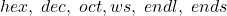, and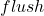are defined in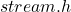header file. The rest are defined in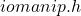header files.

## endl

The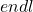introduce a new line or a line feed character. It is similar to C programming language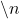character and C++ supports the old line feed.

For example,

cout << "This line use line feed" << endl;
cout << number1 <<  endl << number2 << endl;

You can use it anywhere and a new line character is added automatically.

### Example Program #1

#include <cstdlib>
#include <iostream>
#include <iomanip>
using namespace std;
int main()
{
cout << " This is Accounting Software";
cout << endl;
cout << " The Author is Chang lee.";
cout << endl;

system("PAUSE");
return EXIT_SUCCESS;
}

### Output

This is Accounting Software
The Author is Chang lee.

## setbase()

The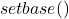manipulator is a manipulator that changes the base of a number to another base value. The C++ language supports following base values:

• oct (Octal = 8)
• dec (Decimal = 10)

Other than the above base converters the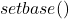can modify base of a variable. The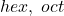, and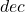manipulator can modify base of input or output numbers.

For example,

int number = 100;
cout << "Hex Value =" << " " << hex << number << endl;
cout << "Octal Value=" << " " << oct << number << endl;
cout << "Setbase Value=" << " " << setbase(16) << number << endl;

The output of the above code is:

Hex Value =  0064
Octal Value =  144
Setbase Value= 0064

### Example Program #2

#include <cstdlib>
#include <iostream>
#include <iomanip>
using namespace std;
int main()
{
//Variable Declaration

int A,B,C;

//Variable Initialization

A = 2078;
B = 3067;

// Computing C

C = A + B;

// Printing Results

cout << "A =" << dec << A << endl;
cout << "B =" << oct << B << endl;
cout << "C = " << setbase(16) << C << endl;

system("PAUSE");
return EXIT_SUCCESS;
}

### Output

A =2078
B =5773
C = 1419

## setw()

The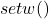is an output manipulator that create whitespace character between two variables. You must specify the an integer value equal to the space required.

setw( int n)

For example,

cout << number1 << number2 << endl;
cout << setw(2) << number1 << setw(5) << number2 << endl;

### Example Program #3:

Your site doesn’t include support for the CodeMirror Blocks block. You can try installing the block, convert it to a Custom HTML block, or remove it entirely.

Install CodeMirror Blocks
Keep as HTML
#include <cstdlib>
#include <iostream>
#include <iomanip>
using namespace std;
int main()
{
//variable declaration

int number1, number2, total;

//variable initialization

number1 = 100;
number2 = 345;

// expression

total = number1 + number2;

//printing output with setw

cout << endl;
cout << endl;
cout << setw(5) << number1 << " + " << setw(5) << number2 << " = "  << setw(6) << total << endl;

system("PAUSE");
return EXIT_SUCCESS;
}

### Output:

100 +   345 =    445

## setfill()

Thefill the whitespaces ofwith a different character. It is an output manipulator like, but the required parameter is a single character. Note that a character is enclosed in between single quotes.

setfill(char ch)

For example,

cout<< setfill('*') << endl;
cout << setw(5) << number1  << setw(5) << number2 << endl;

The output of the above will becharacter between variable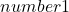and variable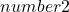.

### Example Program #4:

We will use the aboveexample with slight modifications.

#include <cstdlib>
#include <iostream>
#include <iomanip>
using namespace std;
int main()
{
//variable declaration

int number1, number2, total;

//variable initialization

number1 = 100;
number2 = 345;

// expression

total = number1 + number2;

//printing output with setw

cout << endl;
cout << endl;
cout << setfill('*') << endl;
cout << setw(5) << number1 << " + " << setw(5) << number2 << " = "  << setw(6) << total << endl;

system("PAUSE");
return EXIT_SUCCESS;
}

### Output:

**100 + **345 = ***445

Note the asterisk between variables due tomanipulator.

## setprecision()

The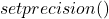is an output manipulator which controls the number of digits to display for a floating-point number after the decimal. The function is defined in the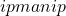header so make sure to include this file in your program.

For example,

float A = 1.34255;
cout << setprecision(3) << A << endl;

The output will be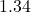.

### Example Program #5:

#include <cstdlib>
#include <iostream>
#include <iomanip>
using namespace std;
int main()
{
//variable declaration
float number1;

//variable initialization

number1 = 34.3358;

//display the number using setprecision()
cout << number1 << endl;
cout << setprecision(2) << number1 << endl;
cout << setprecision(3) << number1 << endl;

system("PAUSE");
return EXIT_SUCCESS;
}

### Output:

34.3358
34
34.3

## ends

The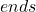adds a null terminating character (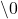) to a string type value. It has no argument like; It just adds a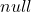.

### Example Program #6:

#include <cstdlib>
#include <iostream>
#include <iomanip>
using namespace std;
int main()
{
// variable declaration

int amount;

// variable initialization

amount = 330;

//display as a string

cout << " \" " << amount << ends << " \" " << endl;

system("PAUSE");
return EXIT_SUCCESS;
}

### Output:

 " 330  "

## ws

The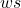is an input stream manipulator that discard any white spaces, therefore, if you have a string with multiple words then the string will display only the first word and trim after that.

### Example Program #7:

#include <cstdlib>
#include <iostream>
#include <iomanip>
using namespace std;
int main()
{
// variable declaration

char name;

cout << "Enter Name" << endl;
cin >> ws;
cin >> name;

//display variable with whitespace

cout << "The Name  is "<< name << endl;

system("PAUSE");
return EXIT_SUCCESS;
}

### Output:

Enter Name
George Land
The Name is George

## Flush

Thefunction clears the output stream. It is an output manipulator means does not work for input stream. The output buffer for console screen is emptied automatically, but the flush is useful in clearing file output buffer after file operations. This function does not take any arguments.

### Example Program #8:

#include <cstdlib>
#include <iostream>
#include <iomanip>
using namespace std;
int main()
{

//using Flush manipulator

cout << "The Beautiful World of Computer Science" << endl;
cout << "Learn Programming First" << endl;
cout.flush();
cout << "Output Buffer Cleared" << endl;

system("PAUSE");
return EXIT_SUCCESS;
}

### Output:

The Beautiful World of Computer Science
Learn Programming First
Output Buffer Cleared

## Setiosflags and Resetiosflags

The input and output stream has flag bits set by default. The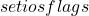and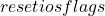helps control the behavior of both streams by modifying the stream flag bits.

Thewill set a flag bit. Alternatively, you can use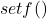function which does the same thing.

setiosflags(long n)

Thewill reset a flag bit, but you may use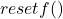function.

setiosflags(long n)

The C++ flags are discussed in more detail in the next lesson.